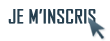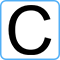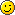IdentifiantMot de passe
Mot de passe oublié ?

Inscrivez-vous gratuitement
pour pouvoir participer, suivre les réponses en temps réel, voter pour les messages, poser vos propres questions et recevoir la newsletterDiscussion :

# Probleme pour afficher des printf dans le main

Sujet :

## C

1.Probleme pour afficher des printf dans le main
salut,
pourquoi lorsque j'ai execute ce programme il ne se passe rien, c'est a dire qu'il n'affiche meme pas les printf dans le main. Qu'est ce qu'il manque ?
 Code : Sélectionner tout - Visualiser dans une fenêtre à part
```12345678910111213141516171819202122232425262728293031323334353637383940414243444546474849505152535455565758596061626364656667#include <stdlib.h>
#include <stdio.h>
#include "NumberArrival.h"
#include "Buffer.h"
//#include "RandomNumber.h"

//Compute the mean delay from the buffer occupancy
double Delay(int S,int N)
{
//calculate mean queue size
double M,delay;
M=(double)S/(double)N;
//printf(" M=%lf\n",M);
//then calculate the queuing delay
delay=(M*681.58)/(1000000.0);
//printf(" delay=%lf\n",delay);
return delay;
}

double SimulationMux(double L)
{
int k,T=8620,A,m=100,S,C;
double MD;
//C stand for buffer occupancy
//S= sum of the queue size
int N=m*T;//simulation for an integer number of periods, i.e., mT time slots
//for timeslot = 1 to infinity,there is 8620 timeslots every 5,875ms(packetization delay)
for(k=0;k<N;k++)
{
if(k==0) { C=0;}
//printf("foo 1");
//figure out how many of the sources generate an arrival NOW
//this is the number of arriving cells - use the equation of evolution
//to figure out the new buffer occupancy
A=ArrivalFromSources(k,L);
//printf("number of arrival = %d \n",A);
C=BufferOccupancy(A,C);
//sum of the queue size :
S+=C;
}
//printf(" S=%d\n",S);
//mean delay
MD=Delay(S,N)+5.875;
//printf("%lf",MD);
return MD;
}

int main()
{
//	int i;
double L,MD;//L=lambda=arrival rate
printf("foo 1");
//	for(i=0;i<=100;i++)
//	{
//		printf("foo 1");
L=0;//		L=0.01*(double)i;
MD=SimulationMux(L);
printf("%lf %lf",L,MD);
//	}

}
//then try to figure out the delay from the above (it can be done!)```
il semble etre parti dans une boucle infiniRépondre avec citation   0  0

2.la variable i n'étant pas déclarée, je ne vois même pas comment il compile !
Elle est commentée...

Décommente i et re-testesRépondre avec citation   0  0

3.printf(...) revient à faire un fprintf(stdout,...) où stdout est un fichier FILE * representant la sortie standard. Les operations sur FILE * sont "bufferisées", c'est à dire que les données sont ecrites rellement dans le fichier lorsque le buffer est plein, ou qu'on le demande, ou qu'on ferme le fichier.
Pour demander explicitement l'ecriture dans le "fichier" (meme si là il s'agit pas de fichier reel), il ya la fonction fflush().

Normalement, si tu mets un fflush(stdout) juste apres ton premier printf, ça devrait s'afficher.Répondre avec citation   0  0

4.ou alors tu ajoute un \n ça mareche aussi ^^Répondre avec citation   0  0

5.Bonjour,

donnes nous la version compilable on pourra y voir plus clair.
Parce que là, il manque déjà le return à la fin du main.Répondre avec citation   0  0

 Actualités FAQ C Tutoriels C Livres C Compilateurs et outils C Sources C GTK+